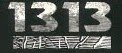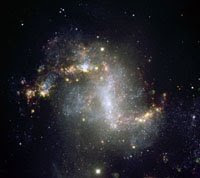## Wednesday, October 29, 2008

### 1313

1313 = 13 x 101.

1313 is an evil number because it has an even number of 1s in its binary expansion: 10100100001.

1313 has two representations as a sum of two squares: 1313 = 172 + 322 = 232 + 282.

1313 is the hypotenuse of two primitive Pythagorean triples: 13132 = 2552 + 12882 = 7352 + 10882.

1313 is a divisor of 1006 - 1.The starburst galaxy NGC 1313 is known as the Topsy-Turvy Galaxy.The Italian poet Giovanni Boaccaccio was born in the year
1313.

Source:
ESO
Image: (c) ESO